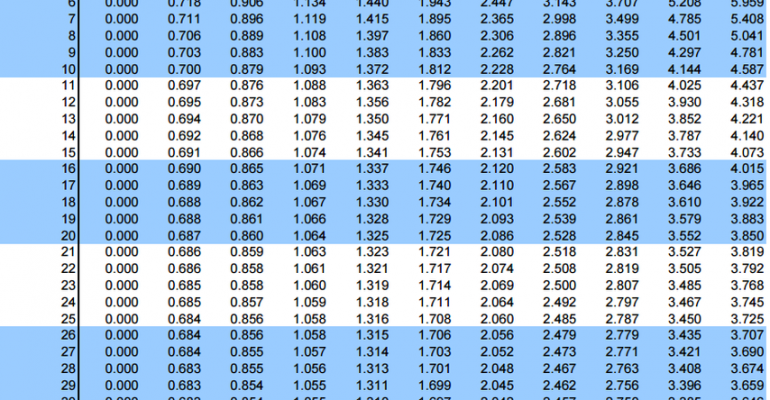Thanks to theidioms.com

## t Table: t Distribution Table with Usage Guide# t Table: t Distribution Table with Usage GuideThe t table or t distribution table is used in statistics when the standard deviation (σ) of a population is not known and the sample size is small, that is, n<30.

The t table is a table that shows the critical values of the t distribution and is given below:

## How to use the t Table or t Distribution Table?

Using the t table is fairly simple during a t-test since you only need to know three values:

• The degrees of freedom of the t-test
• The number of tails of the t-test (one-tailed or two-tailed)
• The alpha level of the t-test (common choices are 0.01, 0.05, and 0.10)

In the t-table, the first column denotes the degrees of freedom of the t-test. So, when you conduct a t-test, you can compare the test statistic from the t-test to the critical value from the t table or t distribution table.

If the test statistic is greater than the critical value found in the table, then you can reject the null hypothesis of the t-test and conclude that the results of the test are statistically significant.

## What is the t-distribution?

The t-distribution or student’s t-distribution is a type of normal distribution that is used for smaller sample sizes where there are more observations towards the mean and fewer observations in the tails.

This means the t-distribution forms a bell curve when plotted on a graph. It is used to find the corresponding p-value from a statistical test that uses the t-distribution such as t-tests and regression analysis.

## When do you use the t table and the z table?

Both t table and z table are used when the population standard deviation is unknown. However, if the sample size is less than 30 then the t table should be used and if not, the z table should be used.

The z table is given below: